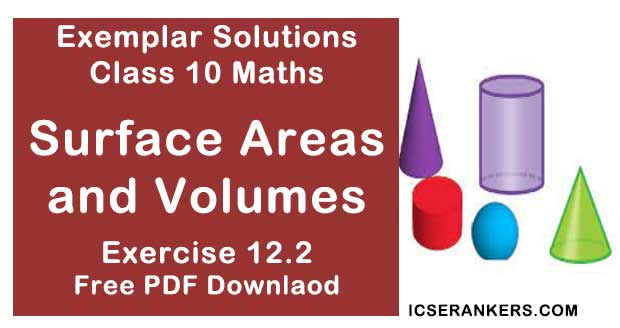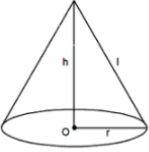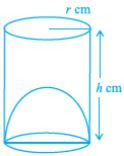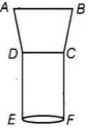# Chapter 12 Surface Areas and Volumes NCERT Exemplar Solutions Exercise 12.2 Class 10 MathsChapter Name NCERT Maths Exemplar Solutions for Chapter 12 Surface Areas and Volumes Exercise 12.2 Book Name NCERT Exemplar for Class 10 Maths Other Exercises Exercise 12.1Exercise 12.3Exercise 12.4 Related Study NCERT Solutions for Class 10 Maths

### Exercise 12.2 Solutions

1. Two identical solid hemispheres of equal base radius r cm are stuck together along their bases. The total surface area of the combination is 6πr2 .

Solution

False
Explanation :
When two hemispheres are joined together along their bases, a sphere of same base radius is formed.
Curved surface Area of a sphere  = 4πr2 .

2. A solid cylinder of radius r and height h is placed over other cylinder of same height and radius. The total surface area of the shape so formed is 4πrh + 4πr2 .

Solution

False
Explanation:
When one cylinder is placed over another, the base of first cylinder and top of other cylinder will not be covered in total surface area.
We know that,
Total surface area of cylinder = 2πrh + 2πr2 h
(Where r = base radius and h = height)
Total surface area of shape formed = 2(Total surface of single cylinder) – 2(Area of base of cylinder) = 2(2πrh + 2πr2) – 2(πr2)
= 4πrh + 2πr2

3. A solid cone of radius r and height h is placed over a solid cylinder having same base radius and height as that of a cone. The total surface area of the combined solid is πr[√(r2 + h2 + 3r + 2h].

Solution

False
Explanation :
When a solid cone is placed over a solid cylinder of same base radius, the base of cone and top of the cylinder will not be covered in total surface area.
Since the height of cone and cylinder is same,
We get,
Total surface area of cone = πrl + πr2 , (where r = base radius and l = slant height)
Total surface area of shape formed = Total surface area of cone + Total Surface area of cylinder – 2(Area of base)Total surface area of cylinder = 2πrh + 2πr2h
(Where r = base radius and h = height)
Total surface area of cylinder = πr(r + l) + (2πrh + 2πr2 ) – 2(πr2 )
= πr2 + πrl + 2πrh + 2πr2 – 2πr2
= πr(r + l + 2h)
= πr [√(r2 + h2 + r + 2h]

4. A solid ball is exactly fitted inside the cubical box of side a. The volume of the ball is
(4/3)πa3.

Solution

False
Explanation :
Let the radius of sphere = r
When a solid ball is exactly fitted inside the cubical box of side a,
We get,
Diameter of ball = Edge length of cube
2r = a
r = a/2
We also know that,
Volume of sphere = (4/3)πr3
Volume of ball = (4/3)π(a/2)3
= (4/3)π(a3/8)
= (1/6)πa3

5. The volume of the frustum of a cone is (1/3)πh[r12 + r22 – r1r2], where h is vertical height of the frustum and r1 , r2 are the radii of the ends.

Solution

False
As volume of the frustum of a cone is  (1/3)πh[r12 + r22 – r1r2]

6. The capacity of a cylindrical vessel with a hemispherical portion raised upward at the bottom as shown in the fig. is (πr3/3)[3h - 2r].Solution

True
We know that, capacity of cylindrical vessel = πr2h - (2/3)πr3
= (πr2/3)[3h - 2r]

7. The curved surface area of a frustum of a cone is πl(r1 + r2 ), where l = √h2 + (r1 + r2 )2 , r1 and r2 are the radii of the two ends of the frustum and h is the vertical height.

Solution

False
We know that,
Curved surface area of a frustum of a cone is πl(r1 + r2),
Where, l = √h2 + (r1 + r2)2 , r1 and r2 are the radii of the two ends  of the frustum and h is the vertical height.

8. An open metallic bucket is in the shape of a frustum of a cone, mounted on a hollow cylindrical base made of the same metallic sheet. The surface area of the metallic sheet used is equal to curved surface area of frustum of a cone + area of circular base + curved surface area of cylinder.

Solution

True
Because the resulting figure is:Here, ABCD is a frustum of a cone and CDEF is hollow cylinder.